# A train begins at the origin and moves at constant speed Vi=10.7 m/s toward another train...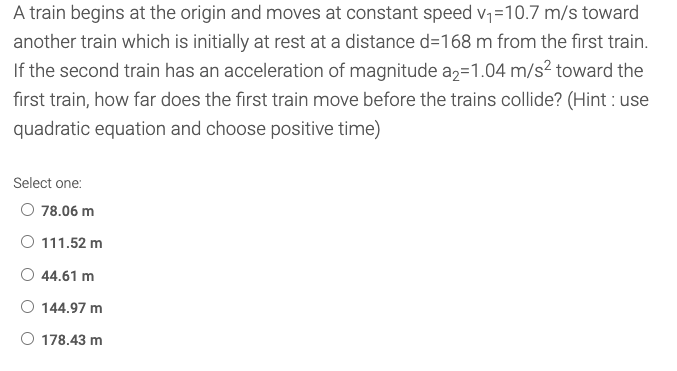A train begins at the origin and moves at constant speed Vi=10.7 m/s toward another train which is initially at rest at a distance d=168 m from the first train. If the second train has an acceleration of magnitude a2=1.04 m/s2 toward the first train, how far does the first train move before the trains collide? (Hint: use quadratic equation and choose positive time) Select one: O 78.06 m O 111.52 m 44.61 m O 144.97 m O 178.43 m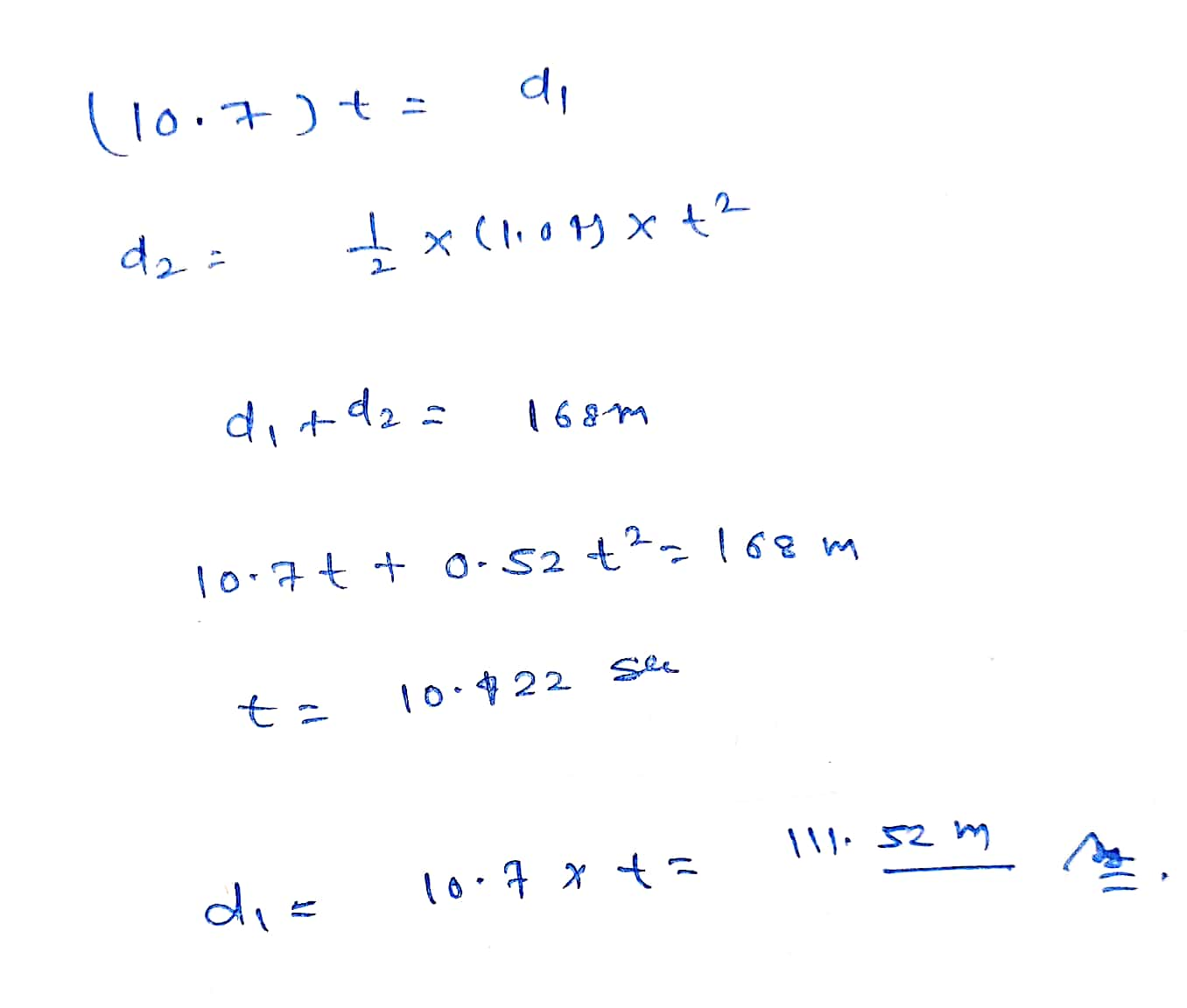##### Add Answer of: A train begins at the origin and moves at constant speed Vi=10.7 m/s toward another train...
Similar Homework Help Questions
• ### A train begins at the origin and moves at constant speed v1=11.7 m/s toward another train...

A train begins at the origin and moves at constant speed v1=11.7 m/s toward another train which is initially at rest at a distance d=152 m from the first train. If the second train has an acceleration of magnitude a2=0.95 m/s2 toward the first train, how far does the first train move before the trains collide? (Hint : use quadratic equation and choose positive time)

• ### A train begins at the origin and moves at constant speed v1=15.2 m/s toward another train...

A train begins at the origin and moves at constant speed v1=15.2 m/s toward another train which is initially at rest at a distance d=164 m from the first train. If the second train has an acceleration of magnitude a2=1.02 m/s2 toward the first train, how far does the first train move before the trains collide? (Hint : use quadratic equation and choose positive time)

• ### A train begins at the origin and moves at constant speed v1=13.1 m/s toward another train...

A train begins at the origin and moves at constant speed v1=13.1 m/s toward another train which is initially at rest at a distance d=264 m from the first train. If the second train has an acceleration of magnitude a2=1.22 m/s2 toward the first train, how far does the first train move before the trains collide? (Hint : use quadratic equation and choose positive time)

• ### A train begins at the origin and moves at constant speed v1=16.1 m/s toward another train...

A train begins at the origin and moves at constant speed v1=16.1 m/s toward another train which is initially at rest at a distance d=108 m from the first train. If the second train has an acceleration of magnitude a2=1.29 m/s2 toward the first train, how far does the first train move before the trains collide? (Hint : use quadratic equation and choose positive time)

• ### A train begins at the origin and moves at constant speed v1=17.8 m/s toward another train...

A train begins at the origin and moves at constant speed v1=17.8 m/s toward another train which is initially at rest at a distance d=232 m from the first train. If the second train has an acceleration of magnitude a2=0.86 m/s2 toward the first train, how far does the first train move before the trains collide? (Hint : use quadratic equation and choose positive time) Select one: 74.15 m 296.59 m 129.76 m 185.37 m 240.98 m

• ### A train begins at the origin and moves at constant speed =18.2 m/s toward another train...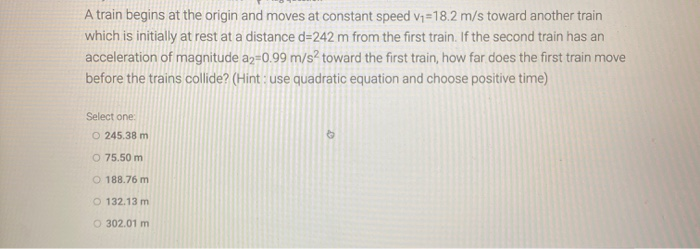A train begins at the origin and moves at constant speed =18.2 m/s toward another train which is initially at rest at a distance d=242 m from the first train. If the second train has an acceleration of magnitude a=0.99 m/s2 toward the first train, how far does the first train move before the trains collide? (Hint: use quadratic equation and choose positive time) Select one 245.38 m O 75.50 m 188.76 m 132.13 m 302.01 m

• ### A train begins at the origin and moves at constant speed V1 =18.2 m/s toward another...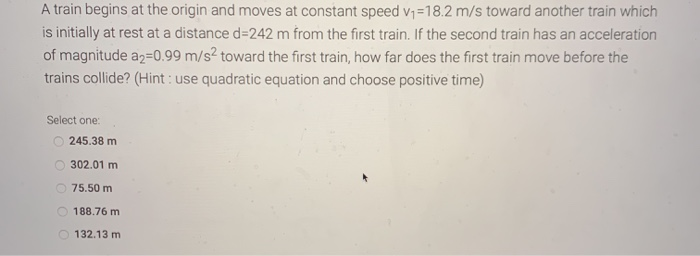A train begins at the origin and moves at constant speed V1 =18.2 m/s toward another train which is initially at rest at a distance d=242 m from the first train. If the second train has an acceleration of magnitude az=0.99 m/s2 toward the first train, how far does the first train move before the trains collide? (Hint: use quadratic equation and choose positive time) Select one: 245.38 m 302.01 m 75.50 m 188.76 m 132.13 m

• ### 8. A model rocket is launched straight upward with an initial speed of 65.0 m/s....continues

8. A model rocket is launched straight upward with an initial speed of 65.0 m/s. It accelerates with a constant upward acceleration of 3.0 m/s2 until its engines stopat an altitude of 150 m.(a) What is the maximum height reached by the rocket? ______meters(b) How long into the flight does the rocket reach maximum height?_______ seconds(c) How long is the rocket in the air? ______ seconds9. Two locomotives approach each other on parallel tracks. Each has a speed of v...

• ### (Dynamics Examination) ist 1, S: 4 If a partide moves along a curve with a constant...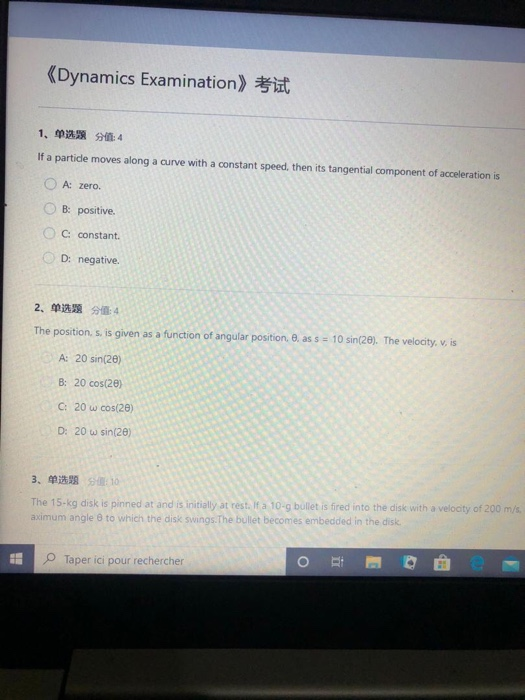(Dynamics Examination) ist 1, S: 4 If a partide moves along a curve with a constant speed, then its tangential component of acceleration is A: zero. B: positive. C. constant D: negative. 2. 单选题的重点 The position, s. is given as a function of angular positione. as s = 10 sin(28). The velocity, v, is A: 20 sin(2) B: 20 cos(20) C: 20 w cos(20) D: 20 w sin(2) 3. S10 The 15-kg disk is pinned at and is initially at...

• ### 2 points SerCP11 33 P My hotes Aak Your Suppose a boat moves at 18.6 m/s...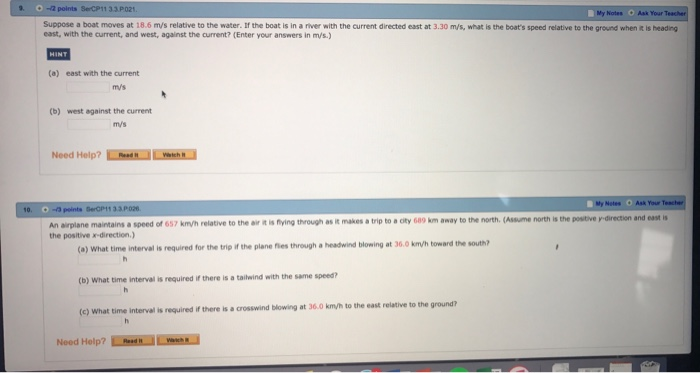2 points SerCP11 33 P My hotes Aak Your Suppose a boat moves at 18.6 m/s relative to the water. If the boat is in a river with the current directed east at 3.30 m/s, what is the boat's speed relative to the ground when it is heading east, with the current, and west, against the current? (Enter your answers in m/s.) (a) east with the current ITV's (b) west against the current m/s Need Help? wwich An airplane maintains...

Free Homework App